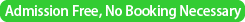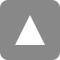﻿ 第50回統計地震学セミナー / The 50th Statistical Seismology Seminar | 統計数理研究所

# 第50回統計地震学セミナー / The 50th Statistical Seismology Seminar

Date&Time
2015年8月4日（火）
/ 4 August, 2015 (Tuesday)　16:00 –Place

/ Coference room 2 (D208) @ The Institute of Statistical Mathematics
Speaker
Yan Y. Kagan
(University of California, Los Angeles (UCLA), Department of Earth and Space Sciences)
Title
" STATISTICS OF EARTHQUAKE FOCAL MECHANISMS "
Abstract

I. Double-couple earthquake source: symmetry and rotation

We analyze earthquake focal mechanisms and their forecast both analytically and statistically. This problem is complex because source mechanisms are tensor-valued variables, thus their analysis requires applying sophisticated mathematical and statistical tools, many of which are not yet fully developed. We describe general and statistical properties of the seismic moment tensor, in particular, its most important form -- the double-couple (DC) mechanism. We establish a method for the analysis of a DC source, based on the quaternion technique, and then apply quaternions for the statistical analysis of earthquake catalogs. The important property of the focal mechanism is its symmetry. We describe the classification of the mechanism symmetry and the dependence of the DC orientation on its symmetry. Four rotations exist in a general case of a DC with the nodal-plane ambiguity, there are two transformations if the fault plane is known, and there is one rotation if the sides of the fault plane are known. A statistical analysis of symmetrical objects has long been the subject of crystallographic texture investigations. We describe the application of crystallographic methods to focal mechanism analysis and consider theoretical statistical distributions appropriate for the DC orientation approximation. Uniform random rotation distributions for various DC sources are discussed, as well as two non-uniform distributions: the rotational Cauchy and von Mises-Fisher. We discuss how the parameters of these rotations can be estimated by a statistical analysis of earthquake source properties in global seismicity using the GCMT catalog. We also show how earthquake focal mechanism orientations can be displayed on the Rodrigues vector space.

II. Statistical earthquake focal mechanism forecasts

In the focal mechanism forecast, the sum of normalized seismic moment tensors within a 1000 km radius is calculated and the P- and T-axes for the predicted focal mechanism are evaluated on the basis of the sum (Kagan and Jackson 1994, JGR). Simultaneously we calculate an average rotation angle between the forecasted mechanism and all the surrounding mechanisms. This average angle shows tectonic complexity of a region and indicates the accuracy of the prediction. Recent interest by CSEP and GEM has motivated some improvements, particularly a desire to extend the previous forecast to polar and near-polar regions. The major problem in extending the forecast is the focal mechanism calculation on a spherical surface. In a modified program focal mechanisms have been projected on a plane tangent to a sphere at a forecast point. A comparison with the old 75S-75N forecast shows that in equatorial regions the forecasted focal mechanisms are almost the same, and the difference in the forecasted focal mechanisms rotation angle is close to zero. However, closer to the 75 latitude degree the difference in the rotation angle is large (around a factor 1.5 in some places). The Gamma-index was calculated for the average focal mechanism moment. A non-zero Index indicates that earthquake focal mechanisms around the forecast point have different orientations. Thus deformation complexity displays itself both in the average rotation angle and in the Index. However, sometimes the rotation angle is close to zero, whereas the Index is large, testifying to a large CLVD presence. Both new 0.5x0.5 and 0.1x0.1 degree forecasts are posted at http://eq.ess.ucla.edu/~kagan/glob_gcmt_index.html

III. Evaluating focal mechanisms forecast skill

We discuss the ways to test the focal mechanism forecast efficiency. We start with several verification methods, first based on ad-hoc, empirical assumptions. However their performance is questionable. In the new work we apply a conventional likelihood method to measure the skill of a forecast. The advantage of such an approach is that an earthquake rate prediction can be adequately combined with a focal mechanism forecast, if both are based on the likelihood scores. This results in a general forecast optimization. To calculate the likelihood score we need to compare actual forecasts or occurrences of predicted events with the null hypothesis that the mechanism's 3-D orientation is random. To better understand the resulting complexities we calculate the information (likelihood) score for two rotational distributions (Cauchy and von Mises-Fisher), which are used to approximate earthquake source orientation patterns. We then calculate the likelihood score for earthquake source forecasts and for their validation by future seismicity data. Several issues need to be explored when analyzing observational results: their dependence on the forecast and data resolution, internal dependence of scores on the forecasted angle, and random variability of likelihood scores. We propose a preliminary solution to these complex problems, as these issues need to be explored by a more extensive theoretical and statistical analysis.

IV. Future focal mechanisms studies

1. Statistical earthquake focal mechanism forecasts, rigorous likelihood methods for evaluating forecast skill.
2. Likelihood analysis of GCMT catalog, including focal mechanisms.
3. Focal mechanism clustering.
4. Collapsing focal mechanism patterns.
5. Influence of Earth surface on focal  mechanisms interaction (Morawiec,Ch8).
6. Integrating Cauchy distribution on Rodrigues space, Morawiec,pp.116-119.
7. Calculating Cauchy and von Mises-Fisher distributions for 120 degrees rotation limit.
8. Investigating the sign-change symmetry of a DC earthquake source.
9. Studies of statistics of earthquake focal mechanisms in the Rodrigues space.
10. Rotation-translation distribution of double-couples as different from arbitrary symmetric deviatoric second-rank tensor.